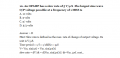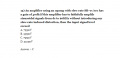# need a help with op amp problems

#### Ghina Bayyat

Joined Mar 11, 2018
135
hi
i have searching on for more problems to help me with the op amp especially with slew ratei couldn't understand the solution or how was it foundalso this problem i didn't know how to solve it

#### Ghina Bayyat

Joined Mar 11, 2018
135

#### upand_at_them

Joined May 15, 2010
877
In my day, if you didn't know an answer you just accepted that you got that one marked incorrect.

#### Ghina Bayyat

Joined Mar 11, 2018
135
In my day, if you didn't know an answer you just accepted that you got that one marked incorrect.
but it is not a homework i found it on the internet

#### ericgibbs

Joined Jan 29, 2010
15,359

Last edited:

#### crutschow

Joined Mar 14, 2008
29,520
•DickCappels

#### Ghina Bayyat

Joined Mar 11, 2018
135
The maximum slew rate of a sinewave is the derivative of the function ia as stated in the problem:

View attachment 214982

Thus you plug the known values into the formula (dV/dt = Vm*2πf) to find the unknown, where dV/dt is the slew-rate, Vm is the peak value of the sinewave, and f is it's frequency.
You do know how to solve for an unknown in such an equation?
of course i know how to solve such equation but actually i don't understand how it was derived
it is easy to find the maximum frequency for a given slew rate and Vm but i don't know i find it complicated to solve for Vm i feel like i'm missing something so i don't understand how this came#### Ghina Bayyat

Joined Mar 11, 2018
135
thank you it is actually helpful but i didn't find what i'm looking for

#### crutschow

Joined Mar 14, 2008
29,520
i know how to solve such equation but actually i don't understand how it was derived
The rate-of-change of a function is found by taking it's derivative.
Thus you take the derivative of the sine function to get its rate-of-change (slew-rate).
The derivative maximum occurs when the COS equals 1.

#### Ghina Bayyat

Joined Mar 11, 2018
135
The rate-of-change of a function is found by taking it's derivative.
Thus you take the derivative of the sine function to get its rate-of-change (slew-rate).
The derivative maximum occurs when the COS equals 1.